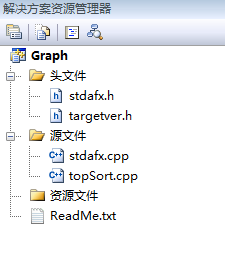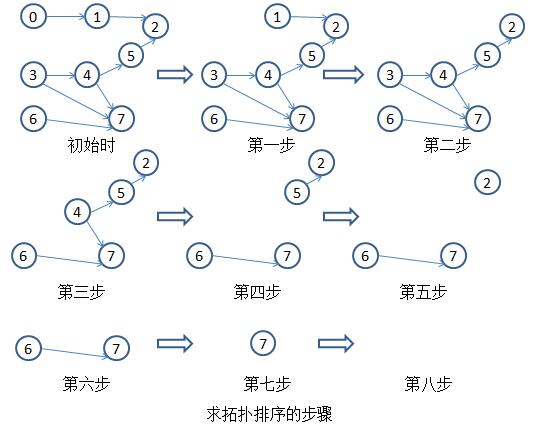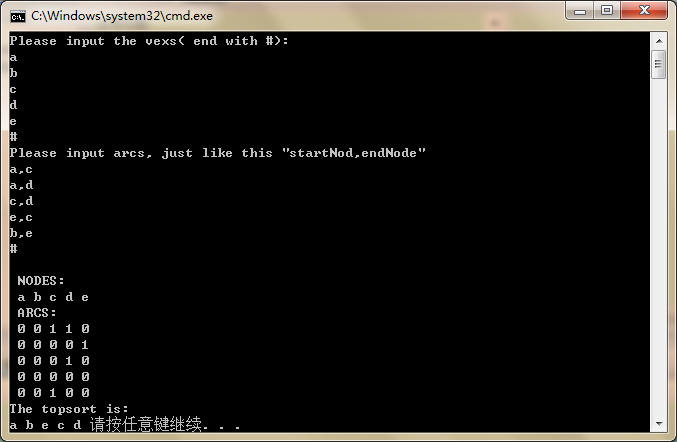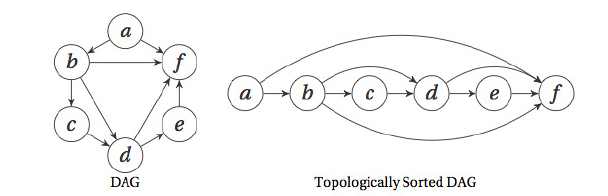• ## 有向图的拓扑排序

万次阅读 多人点赞 2016-03-15 23:01:41
有向图拓扑排序    有向图拓扑排序的基本思想是：首先在有向图中选取一个没有前驱的顶点，将...对于后者，说明有向图中存在环，不能进行拓扑排序。  VS2010下C++ Win32 控制台应用程序，代码如下： #include
                                                                               有向图的拓扑排序

本文取自《数据结构与算法》(C语言版)(第三版)，出版社是清华大学出版社。
本博文作为学习资料整理。源代码是VC++ 6.0上可执行程序，我挪到了VS2010中执行。

在VS2010中新建C++ Win32 控制台应用程序项目，创建结果截图：有向图的拓扑排序的基本思想是：首先在有向图中选取一个没有前驱的顶点，将其输出，从有向图中删除该顶点，并且删除以该顶点为尾的所有有向图的边。重复以上的步骤，直到图中的所有顶点均输出或是图中的顶点均没有前驱为止。对于后者，说明有向图中存在环，不能进行拓扑排序。
以下以AOV(Activity On Vertex Network)网为例，演示求解拓扑排序的步骤：C++ Win32 控制台应用程序，代码如下：topSort.cpp

  #include "stdafx.h"
#include<stdio.h>
#include<string.h>
#define MAXNODE 20
typedef struct
{
char vertex;
}VerNode;

typedef struct
{
}Arc;

typedef struct
{
VerNode vexs[MAXNODE];
Arc arcs[MAXNODE][MAXNODE];
int m_numOfVexs;
}Graph;

int mFind(char aim, VerNode* arry)
{
int pos=-1;
int i=0;
for(i=0; i<MAXNODE; i++)
{
if(aim==arry[i].vertex)
{
pos=i;
return pos;
}
}
return pos;
}

void showGraph(Graph aGraph, int num)
{
int i=0;
int j=0;
printf("\n NODES:\n");
for(i=0; i<num; i++)
{
printf(" %c", aGraph.vexs[i].vertex);
}
printf("\n ARCS:\n");
for(i=0; i<num; i++)
{
for(j=0; j<num; j++)
{
}
printf(" \n");
}
}

//添加顶点
{
aGraph->m_numOfVexs++;
aGraph->vexs[aGraph->m_numOfVexs].vertex=vex;
}

//添加边
void addArcs(Graph* aGraph, char aStart, char aEnd)
{
int p_x=mFind(aStart,aGraph->vexs);
int p_y=mFind(aEnd,aGraph->vexs);
}

//图的初始化
void initGraph(Graph* aGraph)
{
int i=0;
int j=0;
aGraph->m_numOfVexs=-1;
for(i=0; i<MAXNODE; i++)
{
for(j=0; j<MAXNODE; j++)
}
}

int getInDegree(int i, Graph* aGraph)
{
int InDegree=0;
int j=0;
for(j=0; j<aGraph->m_numOfVexs; j++)
return InDegree;
}

int topSort(Graph* aGraph)
{
int i;
int isOK=1;
int vexsIsOut[MAXNODE];
for(i=0; i<aGraph->m_numOfVexs; i++)
{
vexsIsOut[i]=0;
}
while(isOK==1)
{
isOK=0;
for(i=0; i<aGraph->m_numOfVexs; i++)
{
if(vexsIsOut[i]==0&&getInDegree(i,aGraph)==0)
{
int j;
printf("%c ",aGraph->vexs[i].vertex);
vexsIsOut[i]=1;
for(j=0; j<aGraph->m_numOfVexs; j++)
{
}
isOK=1;
}
}
}
for(i=0; i<aGraph->m_numOfVexs; i++)
{
if(vexsIsOut[i]==0)
return 0;
}
return 1;
}

int main(void)
{
char node='a';
char input1='a';
char input2='a';
//将图初始化
Graph g_graph;
initGraph(&g_graph);

//根据用户的输入添加顶点
printf("Please input the vexs( end with #):\n");
while(1)
{
if(node=='#')
break;
if(scanf("%c,",&node)==1)
{
if(node=='\n')
continue;
}
}

//根据用户的输入添加边
printf("Please input arcs, just like this \"startNod,endNode\" \n");
while(1)
{
if(input1=='#')
break;
if(scanf("%c,%c",&input1, &input2)==2)
{
if(input1=='\n'||input2=='\n')
continue;
}
}

//输出图
showGraph(g_graph, g_graph.m_numOfVexs);

printf("The topsort is: \n");
if(topSort(&g_graph)==0)
printf("There is a circle in the graph!! \n");
return 0;
}


Ctrl+F5执行topSort.cpp代码，结果如下图：展开全文图算法 C
• 给定一个n个点m条边的有向图，点的编号是1到n，图中可能存在重边和自环。 请输出任意一个该有向图拓扑序列，如果拓扑序列不存在，则输出-1。 若一个由图中所有点构成的序列A满足：对于图中的每条边(x, y)，x在A...
思路：
用数组模拟链表，首先如果能用拓扑排序则其一定是有向无环图。
所以我们应该先找到其入度为0的点。然后将其入队，并将与此点相连的边全部删去。最后当队尾指针是n-1时说明一共入队n个元素说明此图是有向无环图。存在拓扑排序。
注意：h[i]中存储的是元素在e[]中的下标。
问题描述
给定一个n个点m条边的有向图，点的编号是1到n，图中可能存在重边和自环。
请输出任意一个该有向图的拓扑序列，如果拓扑序列不存在，则输出-1。
若一个由图中所有点构成的序列A满足：对于图中的每条边(x, y)，x在A中都出现在y之前，则称A是该图的一个拓扑序列。
输入格式
第一行包含两个整数n和m
接下来m行，每行包含两个整数x和y，表示存在一条从点x到点y的有向边(x, y)。
输出格式
共一行，如果存在拓扑序列，则输出任意一个合法的拓扑序列即可。
否则输出-1。
数据范围
1≤n,m≤105
输入样例：
3 3
1 2
2 3
1 3
输出样例：
1 2 3
代码
#include<iostream>
#include<cstring>
using namespace std;
const int N = 100010;
int e[N], ne[N], h[N], idx = 0;
int q[N], d[N];
int n = 0, m = 0;
e[idx] = b, ne[idx] = h[a], h[a] = idx++;
}

bool toposort()
{

int hh = 0, tt = -1;
for (int i = 1; i <= n; ++ i){
if (!d[i]){
q[++ tt] = i;
}
}
while(hh <= tt){
int t = q[hh++];
for(int i = h[t]; i != -1; i = ne[i]){
d[e[i]]--;
if(!d[e[i]])
q[++tt] = e[i];
}
}
return tt == n-1;
}

int main(){
cin >> n >> m;
int a, b;
memset(h, -1, sizeof h);
for(int i = 0; i < m; ++i){
cin >> a >> b;
d[b]++;
}
if(toposort()){
for(int i = 0; i <= n - 1; ++i){
cout << q[i] << " ";
}
}else{
cout << "-1" << endl;
}
return 0;
}

原题链接


展开全文链表 c++ 算法
• ## 有向图及拓扑排序

万次阅读 2018-10-20 23:06:53
有向图 在无向图中，边没有方向，两条边之间的顶点是单向可达...对有向图的结构定义如下： #include <map> #include <forward_list> using namespace std; struct DirectedGraph { size_t V, E;...
有向图

在无向图中，边没有方向，两条边之间的顶点是单向可达的，而有向图的边是单向的。虽然边的性质不同，但我们仍然可以用邻接表来表示有向图。对有向图的结构定义如下：

#include <map>
#include <forward_list>

using namespace std;

struct DirectedGraph
{
size_t V, E; //V表示顶点数，E表示边数
};


有向图在计算机中有广泛的应用，如任务调度条件、网络等。有向图的顶点之间的联系是描述现实世界的有利工具，如计算机的任务调度系统中，根据多任务之间的先后关联需要给出任务的执行顺序，拓扑排序就是可以得到这一顺序的算法。

拓扑排序

给定一幅有向图，将所有的顶点排序，使得所有的有向边均从排在前面的元素指向排在后面的元素（或者说明无法做到这一点）。还是以任务调度系统为例，假设一个任务x必须在任务y之前完成，任务y必须在任务z之前完成，任务z又必须在任务x之前完成，这样的问题肯定是无解的。也就是说，拓扑排序能得出结果的前提是图必须是有向无环图（Directed Acyclic Graph，DAG），即一幅不含有环路的有向图。

一种拓扑排序的思想是基于深度优先搜索的顶点排序。它的基本思想是深度优先搜索会沿着开始顶点一直向下搜索，且正好只会访问每个顶点一次。基于深度优先搜索的拓扑排序基于一个重要命题：一幅有向图的拓扑顺序即为所有顶点的逆后序排列。所谓逆后序遍历即在路径达到最大深度后再保存（打印）顶点，得到后序遍历，将其逆向输出即可。

下面是基于深度优先搜索来得到拓扑排序后的顶点顺序（默认无环，因此未给出判断是否存在有向环的代码）：

void dfs(DirectedGraph &g, vector<int> &visited, stack<int> &st, int v)
{
visited[v] = 1;
{
if (!visited[v])
{
dfs(g, visited, st, i);
}
}
st.push(v);
}

void TopologicalSort(DirectedGraph &g, int v)
{
stack<int> st;
vector<int> visited(g.V);
dfs(g, visited, st, v);
while (!st.empty())
{
cout << st.top() << " ";
st.pop();
}
}

另一种思路是得到所有顶点的入度，循环执行以下两个步骤直到不存在入度为0的顶点：

（1）选择一个入度为0的顶点，输出

（2）将该顶点其出边全部删除，同时更新出边所到达顶点的入度

这种算法不需要太多代码判断是否存在有向环，只要最后输出的顶点数小于有向图的顶点数就说明了存在有向环。

bool TopologicalSort(DirectedGraph &g, vector<int> &in_degree)
{
queue<int> qu;
int cnt = 0;
for (auto ite = in_degree.begin(); ite != end(); ++ite)
{
if (0 == *ite)
{
qu.push(*ite);
}
}
while (!qu.empty())
{
int v = qu.front();
qu.pop();
cout << v << " ";
++cnt;
for (const int &i : g.adj.at(v))
{
if (0 == --in_degree[i])
{
qu.push(i);
}
}
}
return cnt < V ? false : true;
}


展开全文• 这种用顶点表示活动，用弧表示活动间的优先关系的有向图称为顶点表示活动的网（Activity On Vertex Network），简称AOV-网。 按照我的理解是：AOV-网是不带权值且没有回路的有向图。 完整代码如下： #include <...
这里使用我随便画的例子：这种用顶点表示活动，用弧表示活动间的优先关系的有向图称为顶点表示活动的网（Activity On Vertex Network），简称AOV-网。
按照我的理解是：AOV-网是不带权值且没有回路的有向图。
完整代码如下：
#include <stdio.h>
#include <stdlib.h>
#include <string.h>
#define MVNum 100 //最大顶点数
#define MAXSIZE 100 //最大栈容量

void Interrupt(void)//创建一个中断函数
{
while(1)//用于检测换行符，使函数脱离scanf的连续输出
if(getchar()=='\n')
break;
}

//导入栈定义
typedef struct
{
int data[MAXSIZE];//静态顺序栈可用的最大容量
int top;//栈顶
}SqStack;

void InitStack(SqStack &S)//栈的初始化
{
S.top = -1;//静态顺序栈中，使S.top=-1便是对栈的初始化
}

int Push(SqStack &S,int e)//进栈
{
if(S.top==MAXSIZE-1)//判断栈是否为满
{
printf("栈满！\n");
return 0;
}
S.data[++S.top]=e;//S.top自加一，使S.top=0,使输入的e值导入栈中
return 0;
}

bool StackEmpty(SqStack S)//栈的判空操作
{
if(S.top == -1)//若栈为空返回true，否则为false
return true;
else
return false;
}

int Pop(SqStack &S)//使栈顶元素出栈，并返回栈顶元素，且栈长减一
{
if(StackEmpty(S))//首先先判断栈是否为空
{
printf("栈空!\n");
return -1;
}
else
return S.data[S.top--];
}

//导入邻接矩阵定义
typedef struct ArcNode
{
struct ArcNode *nextarc;//该向下一条边的指针
}ArcNode;
typedef struct VNode//顶点信息
{
char data;//顶点名称
ArcNode *firstarc;//指向第一条依附该顶点的边的指针
typedef struct//邻接表
{
int vexnum,arcnum;//图的当前顶点数和边数
}ALGraph;

void InitGraph(ALGraph &G)//图的初始化
{
int i;
for(i=0;i<MVNum;i++)
G.vertices[i].firstarc = NULL;//使所有的第一个结点都置空，也就是后面设定的尾指针的判空操作
}

void CreateGraph(ALGraph &G)//图的创建
{
int i;//记录次数
char a;//顶点变量
printf("请输入顶点数和边数:");
scanf("%d %d",&G.vexnum,&G.arcnum);//顶点数和边数的赋值
Interrupt();//该函数用于检测并吸收换行符
printf("请输入顶点名称（连续输入）：");
for(i=0;i<G.vexnum;i++)//利用循环输入图中顶点名称
{
scanf("%c",&a);
G.vertices[i].data = a;//第i个顶点的命名
}
Interrupt();//该函数用于检测并吸收换行符
char b,c;//顶点变量
int w,j,k;//w为权值变量，j和k是用来记录次数的
for(i=0;i<G.arcnum;i++)//利用循环输入所有边的两个顶点和权值
{
printf("请输入边的两个顶点:");
scanf("%c %c",&b,&c);//输入
Interrupt();//该函数用于检测并吸收换行符
for(j=0;j<G.arcnum;j++)//该操作为书上的函数LocateVex操作
{
if(G.vertices[j].data == b)//找到输入的顶点b的位置
break;
}
for(k=0;k<G.arcnum;k++)
{
if(G.vertices[k].data == c)//找到输入的顶点c的位置
break;
}
ArcNode *p1;//创建两个野结点
p1 = (ArcNode*)malloc(sizeof(ArcNode));
p1->nextarc = G.vertices[j].firstarc;//类似于头插法
G.vertices[j].firstarc = p1;//并使头结点永远放在第一位
}
}

void InputGraph(ALGraph G)//邻接表的输出
{
int i,j;//记录次数
ArcNode *p;//用于遍历链表
printf("邻接表为：\n");
for(i=0;i<G.vexnum;i++)//利用循环输出
{
printf("%c",G.vertices[i].data);
p = G.vertices[i].firstarc;
while(p)//当p为空时，结束循环
{
p = p->nextarc;//p指向p的下一个结点
}
printf("\n");
}
printf("\n");
}

void FindInDegree(ALGraph G,int indegree[])//拓扑排序中所用到的各顶点入度函数
{
int i,j;
ArcNode *p;//创建一个指针
for(i=0;i<G.vexnum;i++)//数组初始化
indegree[i] = 0;
for(i=0;i<G.vexnum;i++)//遍历整个邻接表
{
p = G.vertices[i].firstarc;//指针指向第i个结点的firstarc
while(p != NULL)//判断p是否为空 ，这里和拓扑排序函数中的刚好相反
{
indegree[j]++;
p = p->nextarc;
}
}
}

bool TopologicalSort(ALGraph G,int topo[])//拓扑排序
{
int i,m,k;
int indegree[G.vexnum];
FindInDegree(G,indegree);//求各顶点入度函数
SqStack S;
InitStack(S);//引入栈
for(i=0;i<G.vexnum;i++)//遍历各顶点
{
if(indegree[i] == 0)//入度为0的顶点进栈
Push(S,i);
}
m = 0;//对输出顶点计数，初始化为0
ArcNode *p;
while(StackEmpty(S) != true)//栈S非空
{
i = Pop(S);//出栈，并赋值给i
topo[m] = i;//将Vi保存在拓扑排序数组topo中
m++;//对输出顶点计数
p = G.vertices[i].firstarc;//p指向Vi的第一个邻接点
while(p != NULL)
{
indegree[k]--;//Vi的每个邻接点的入度减1
if(indegree[k] == 0)//若入度减为0，则入栈
Push(S,k);
p = p->nextarc;//p指向Vi下一个邻接结点
}
}
if(m<G.vexnum)//该有向图有回路
return false;
else
return true;
}

int main()
{
ALGraph G;
InitGraph(G);//初始化
CreateGraph(G);//邻接表的创建
InputGraph(G);//邻接表的输出
int i,a[G.vexnum];
if(TopologicalSort(G,a)) //调用拓扑排序，若没有回路函数为true，否则false
for(i=0;i<G.vexnum;i++)//利用循环打印拓扑后的值
printf("%c  ",G.vertices[a[i]].data);
else
printf("有回路！");
return 0;
}


结果演示：（完）


展开全文数据结构 c语言
• #include #include #include #define MAXN 10 struct ArcNode { int to; struct ArcNode *next; }; int n,m; //顶点个数和边数 struct ArcNode * List[MAXN]; int count[MAXN]; char output;... i
• 有向图拓扑排序拓扑排序是可以用图模拟的另一种操作。它可以用于表示一种情况，即某些项目或事件必须按照特定的顺序排列或发生。 如：课程的优先关系 有向图： 图可以表示这类关系，然而，图需要有一种前面...
• 1.有向图拓扑排序 2.关键路径  2.1.邻接链表存储AOE网  2.1.1拓扑排序： bool TopologicalOrder()： 2.1.2关键路径：bool CriticalPath() 求关键路径的完整代码与测试用例： 无向图、有向图判环...AOE关键路径
• 一个有向图拓扑排序（Topological sort 或 Topological ordering）是根据其有向边从顶点U到顶点V其所有顶点的一个线性排序 举个例子：让一个拓扑排序的图中的所有顶点代表某项要执行的任务组合，那么它的边就...python 数据结构
• 力扣题解，利用有向图拓扑排序解决课程表的课程依赖问题dfs bfs leetcode
• 问题描述：对于一个有n个节点的有向图，判断其有无回路，如果没有请输出一条拓扑排序路径。采用邻接链表存储 输入采用文件格式，如下 //建立有向图的文件输入格式为 //顶点个数n //顶点0出度k1 m1 m2 … mk //...
• 力扣题解，210. 课程表 II，利用有向图拓扑排序解决课程表的课程依赖问题。算法 dfs bfs leetcode强连通分量 最短路径 算法
• **拓扑排序**拓扑排序：给一幅有向图，把所有的顶点排序，使所有的有向边都从 排在前面的顶点 指向排在后面的顶点。（或者证明不能做到，比如有环） 拓扑排序可以用来解决优先级调度的问题。（前后顶点有依赖关系）...
•dfs C++
• 给定一个有向图G=（V,E），将之进行拓扑排序，如果图有环，则提示异常。 要想实现图的算法，如拓扑排序、最短路径……并运行看输出结果，首先就得构造一个图。由于构造图的方式有很多种，这里假设图的数据存储在一...
• 一、以下为有向图 经过拓扑排序如下： 二、实现代码，首先实现拓扑排序如下： package com.tool.wpn.quicksort; ... * 图-有向图拓扑排序 */ public class GraphTopo { private finjava 数据结构 算法
• 拓扑排序：有向无环图（拓扑图） 拓扑序列是顶点活动网中将活动按发生的先后次序进行的一种排列 前提： 有向无环图→\rightarrow→ ...有向图的拓扑序列：https://www.acwing.com/problem/content/850/ #include<
• 拓扑排序一、有向图的拓扑序列二、代码实现 一、有向图的拓扑序列 给定一个n个点m条边的有向图，点的编号是1到n，图中可能存在重边和自环。 请输出任意一个该有向图的拓扑序列，如果拓扑序列不存在，则输出-1。 若...
• 在图论中，如果一个有向图无法从某个顶点出发经过若干条边回到该点，则这个图是一个有向无环图（DAG图）。而提到DAG，就差不多会联想到拓扑排序拓扑排序是指由某个集合上的一个偏序得到该集合上的一个全序的操作。...数据结构
• 拓扑排序G中所有节点的一种线性次序，该次序满足以下条件：如果G包含边(u,v)，则节点u在拓扑排序中处于节点v的前面。 在实际生活中有很多应用需要用有向无环来指明事件的优先次序。扫搜索 算法
• 拓扑排序有向图中全部顶点的一种线性排序，只有有向图中入度为0的顶点才能成为拓扑排序的第一个顶点，不断重复地寻找入度为0的顶点，即可得到拓扑排序结果，具体方法可以通过深度优先搜索或广度优先搜索来解决有向...dfs bfs 算法
• 如果图中存在环(回路)，那么该图不存在拓扑排序，在这里我们讨论的都是无环的有向图。 什么是拓扑排序 一个例子 对于一部电影的制作过程，我们可以看成是一个项目工程。所有的工程都可以分为若干个"活动"...
• (1)、有向图，边是有方向的。 邻接矩阵表示方法上下三角形是不对称的。在添加一条边是只需要一条语句， ...(2)、有向图的算法-拓扑排序 有向图的应用，某些项目或者事件必须按照特定的顺序排序或发生。graph class output string date...